Question

A charge of 2.00 μC flows onto the plates of a capacitor when it is connected to a 12.0-V potential source. What is the minimum amount of work that must be done in charging this capacitor?

1.thugiang

To develop this problem we will apply the concept of energy conservation. For which the work carried out must be equivalent to the potential energy stored on the capacitor. We will start by finding the capacitance to later be able to calculate the energy and therefore the work in the capacitor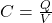Here,

C = Capacitance

V = Potential difference between the plates

Q = Charge between the capacitor plates

At the same time the energy stored in the capacitor can be defined as,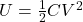We will start by finding the value of the capacitance, so we will have to,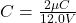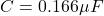Finally using the expression for the energy we have that,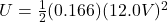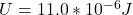Therefore the minimum amount of work that must be done in charging this capacitor is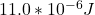2.RobertKer

12×10⁻⁶ J.

Explanation:

The minimum amount of work that must be done in other to charge a capacitor = equal to the energy stored in a capacitor.

The Formula for the energy stored in a capacitor is given as,

E = 1/2QV………………….. Equation 1

Where E = Energy stored in a capacitor, Q = Charge on the capacitor, V = Potential difference connected across the plates of the capacitor.

Given: Q = 2×10⁻⁶ C, V = 12.0 V

Substitute into equation 1

E = 1/2(2×10⁻⁶)(12.0)

E = 12×10⁻⁶ J.

Hence the minimum amount of work that must be done in charging the capacitor = 12×10⁻⁶ J.